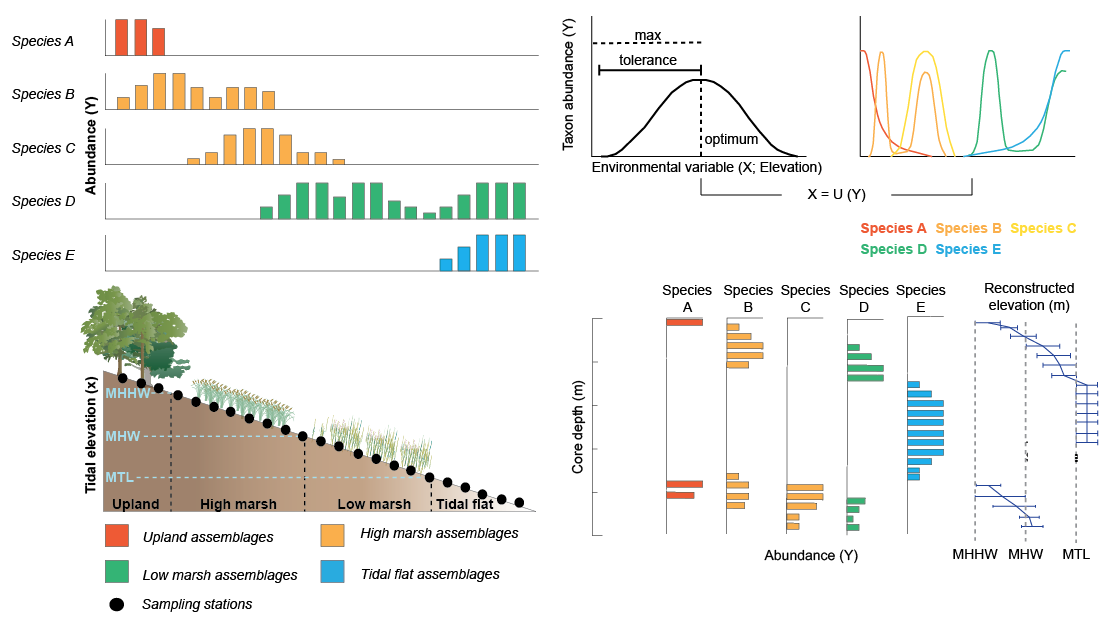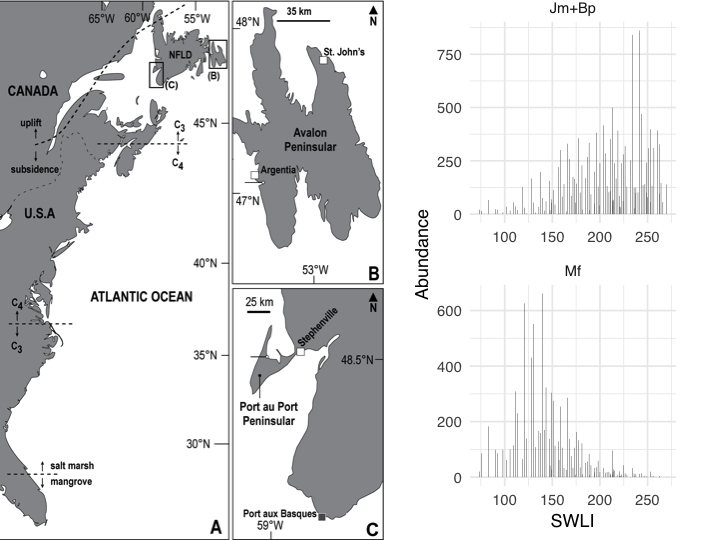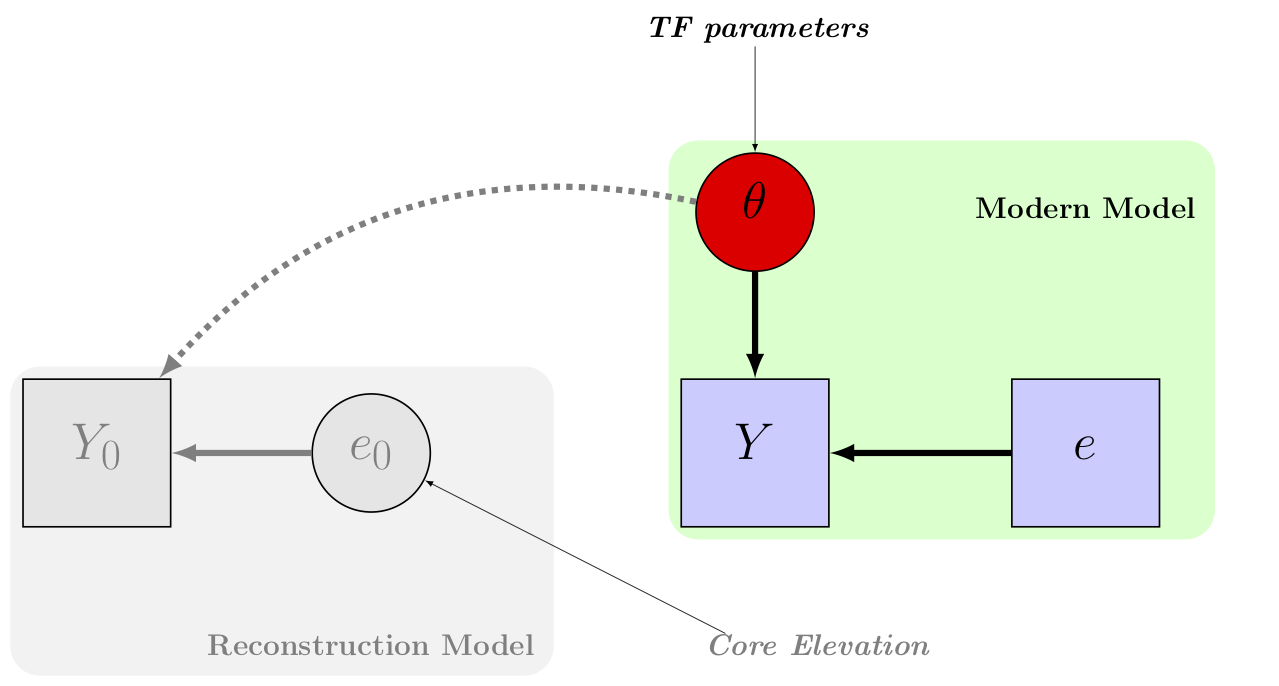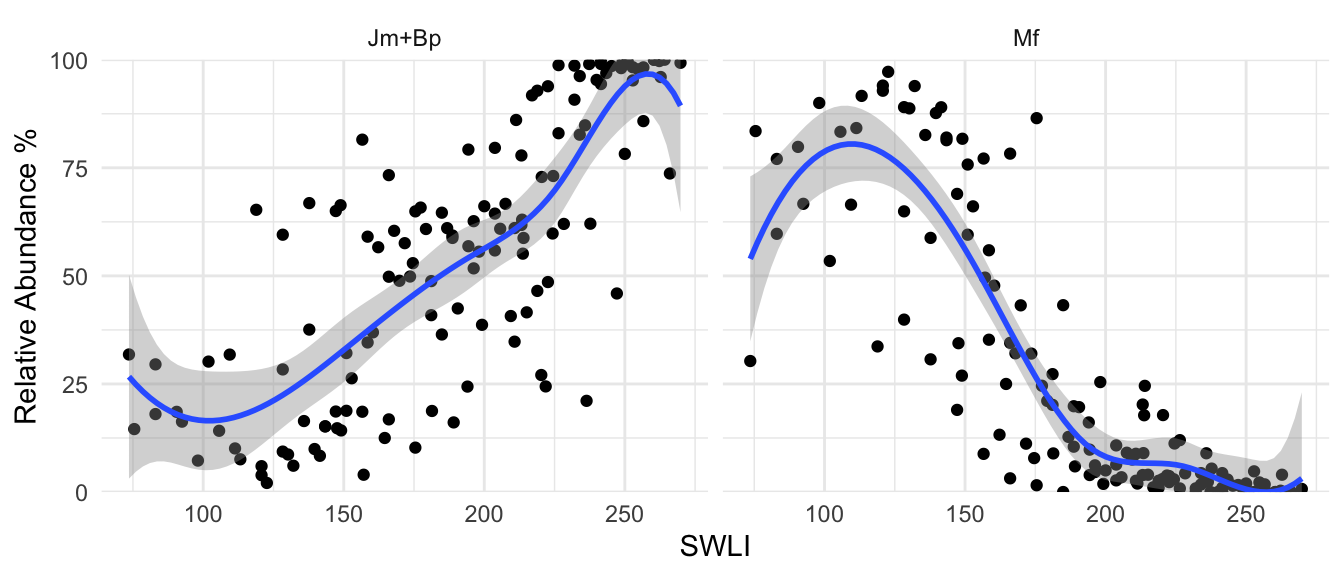January 14th 2021

## Reconstructing Relative Sea Level## Case Study: Newfoundland## A Bayesian Transfer Function (BTF)

$$\underbrace{P(parameters|Data)}_\text{Posterior}\propto \underbrace{P(Data|parameters)}_\text{Likelihood}\underbrace{P(parameters)}_\text{Prior}$$## The Process Model

• For modelling puposes we consider a latent species response variable

• Each latent species variable has a functional relationship with elevation:

${\bf s}_j = f_{\theta_j}(\text{elevation}) + {\bf\epsilon}_j$

• f is a penalised-spline function and $${\bf \theta}_j$$ is a vector of parameters controlling the shape of the spline for species $$j$$.

• $$\epsilon_{ij} \sim N(0, \sigma_j^2)$$ and is added to account for overdispersion (i.e, the data here are likely to exhibit more variation than may be capturped with the data model).

## The Data Model

• The observed species abundances are multinomial

$(y_{i1},....,y_{iM}) \sim Multi(p_{i1},....,p_{iM}, N_i)$

• The probabilites of the multinomial distribution are estimated as a function of the latent species variable via a softmax transformation where

${p}_{ij} = \frac{e^{s_{ij}}}{\sum_{j=1}^M e^{s_{ij}}}$

## Species Response Curves## A Bayesian Transfer Function (BTF)

$$\underbrace{P(parameters|Data)}_\text{Posterior}\propto \underbrace{P(Data|parameters)}_\text{Likelihood}\underbrace{P(parameters)}_\text{Prior}$$